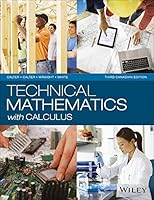# Technical Mathematics with Calculus, 3rd Canadian Edition0 Reviews
2016
1024 pages

## Book Description

A non-theoretical book packed with applications in ! This invaluable book is renowned for its many, fully-worked examples and numerous applications. Throughout, effective illustrations make the material clear and easy to understand.

Chapter 1 Numerical Computation
Chapter 2 Introduction to
Chapter 3 Simple Equations and Word Problems
Chapter 4 Functions
Chapter 5
Chapter 6 Geometry
Chapter 7 Right Triangles and Vectors
Chapter 8 Factors and Factoring
Chapter 9 Fractions and Fractional Equations
Chapter 10 Systems of Linear Equations
Chapter 11 Determinants
Chapter 12 Matrices
Chapter 15 Oblique Triangles and Vectors
Chapter 16 Radian Measure, Arc Length, and Circular Motion
Chapter 17 Graphs of the Trigonometric Functions
Chapter 18 Trigonometric Identities and Equations
Chapter 19 Ratio, Proportion, and Variation
Chapter 20 Exponential and Logarithmic Functions
Chapter 21 Complex Numbers
Chapter 22 Analytic Geometry
Chapter 23 Binary, Hexadecimal, Octal, and BCD Numbers
Chapter 24 Inequalities and Linear Programming
Chapter 25 Sequences, Series, and the Binomial Theorem
Chapter 26 Introduction to and Probability
Chapter 27 Derivatives of Functions
Chapter 28 Applications of the Derivative
Chapter 29 Applied Applications of the Derivative
Chapter 30 Integration
Chapter 31 Applications of the Integral
Chapter 32 More Applications of the Integral
Chapter 33 Derivatives of Trigonometric, Logarithmic, and Exponential Functions
Chapter 34 Methods of Integration
Chapter 35 Differential Equations
Chapter 36 Solving Differential Equations by the Laplace Transform and by Numerical Methods
Chapter 37 Infinite Series

## Book Details

• Title: Technical Mathematics with Calculus, 3rd Canadian Edition
• Author: , ,
• Length: 1024 pages
• Edition: 1
• Language: English
• Publisher:
• Publication Date: 2016
• ISBN-10: 1118962141
• ISBN-13: 9781118962145